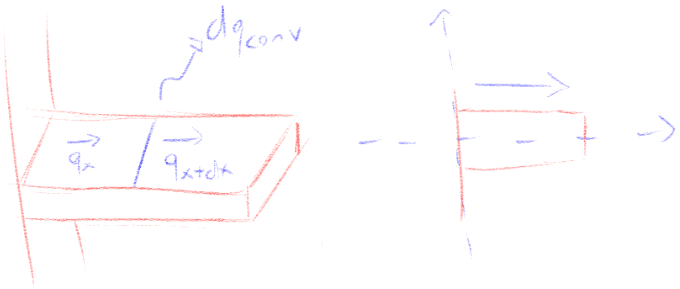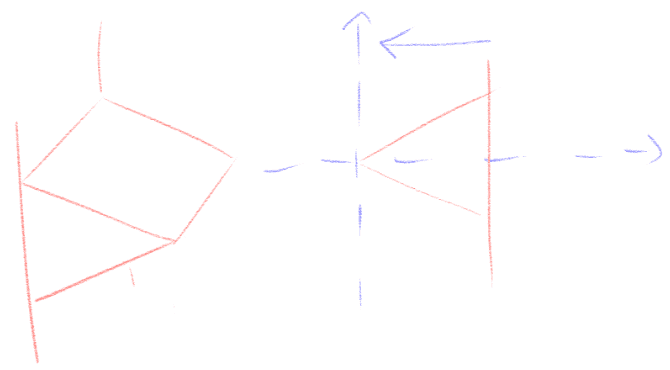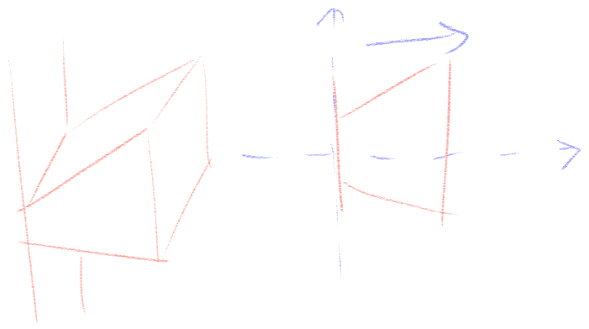Heat transfer direction in fins

Homework Statement

I'm unsure of what exactly is changing the heat transfer direction in the triangular fin.

Homework Equations

$$q_{x} = -kA(x)\frac{dT(x)}{dx} (1)$$
$$q_{x+dx} = -kA(x)\frac{dT(x)}{dx} - k\frac{d}{dx}[A(x)\frac{dT(x)}{dx}] (2)$$
$$dq_{conv} = h(x)dS(x)P[T(x) - T_{∞}] (3)$$
A = cross-sectional area m²
q = heat transfer rate W
h = convection heat transfer coefficient W/m²K
k = thermal conductivity W/mK
T = temperature K
dq = convection heat transfer rate
dS = surface area of the differential element
Tb = base surface temperature K
A0 = base area
L = length

The Attempt at a Solution

Energy balance:
$$q_{x} = dq_{conv} + - q_{x+dx} (4)$$
Substitute (1), (2) and (3) in (4):
$$\frac{d}{dx}[A(x)\frac{dT(x)}{dx}] - \frac{h(x)dS(x)}{kdx}[T(x) - T_{∞}] (5)$$
$$X = \frac{x}{L}$$
$$\theta(X) = \frac{T(X) - T_{∞}}{T_{b} - T_{∞}}$$
$$K(X) = \frac{A(X)}{A_{0}}$$
$$W(X) = \frac{h(X)dS(X)}{P_{0}h_{av}dX}$$
$$M = mL$$
$$m² = \frac{h_{av}P_{0}}{kA_{0}}$$
$$\frac{dS(X)}{dX} = p(X)$$
Substitute into (5) and rearrange:
$$\frac{d}{dX}[K(X)\frac{d\theta(X)}{dX}] - W(X)M²\theta(X) = 0 (6)$$

Rectangular profile:A(X) = A0, K(X) = 1, W(X) = 1
$$\frac{d}{dX}[\frac{d\theta(X)}{dX}] - M²\theta(X) = 0$$

Triangular profile:A(X) =/= A0, K(X) = ~ X, W(X) = 1
$$\frac{d}{dX}[X\frac{d\theta(X)}{dX}] - M²\theta(X) = 0$$

I did the energy balance thinking of the heat going from left to right like it's shown on the rectangular fin, but on the triangular fin it goes on the opposite direction. Which is fine for what I want to do, but why exactly does this happen? Due to the equations involved the heat will only move from a bigger to an equal or smaller area? Which adjustments would I have to make to change the heat transfer direction and make a fin like this:jambaugh